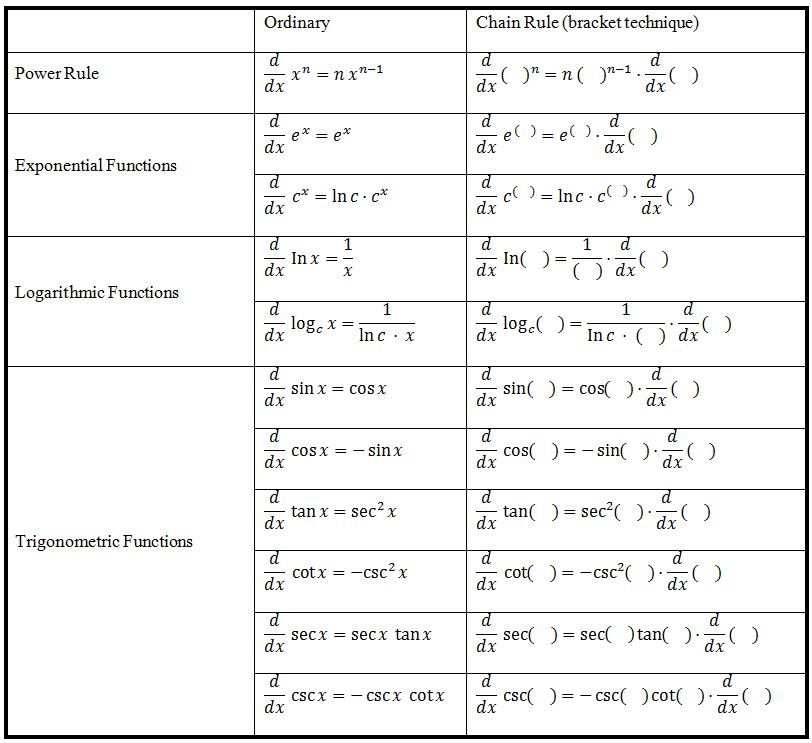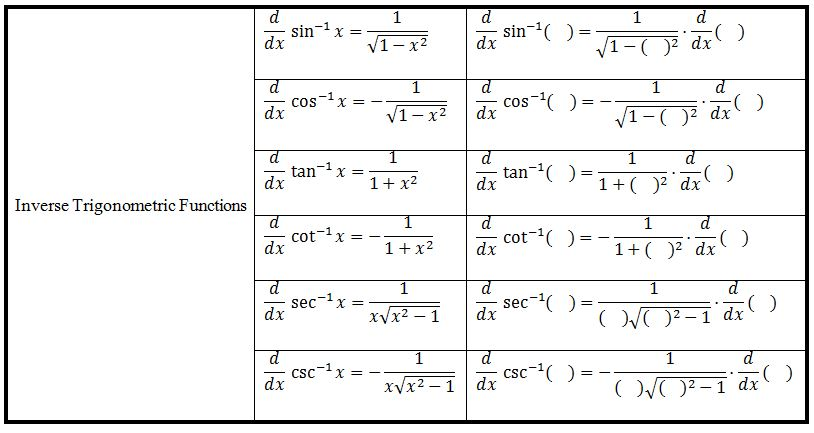# Chain rule

### Chain rule

Chain Rule appears everywhere in the world of differential calculus. Whenever we are finding the derivative of a function, be it a composite function or not, we are in fact using the Chain Rule. In this section, we will learn about the concept, the definition and the application of the Chain Rule, as well as a secret trick – "The Bracket Technique".

#### Lessons

Chain Rule
if: $y = \;f\left( {\;\;\;\;\;\;\;} \right)$
then: $\frac{{dy}}{{{d}x}} = f'\left( {\;\;\;\;\;\;\;} \right)\cdot\frac{{d}}{{{d}x}}\left( {\;\;\;\;\;\;\;\;} \right)$

Differential Rules• Introduction
Introduction to Chain Rule
• "bracket technique" explained!
exercise: $\frac{d}{dx}x^{10}$ VS. $\frac{d}{dx}(x^5+4x^3-6x+8)^{10}$

• 1.
Differentiate: Polynomial Functions
$\frac{d}{dx} (2x-1)^3$

• 2.
Differentiate: Rational Functions
a)
$\frac{d}{dx} \frac{1}{(4x^3+7)^{10}}$

b)
$\frac{d}{dx}- \frac{5}{\sin ^2x}$

• 3.
a)
$\frac{d}{dx} \sqrt{x^3+4x^2-9}$

b)
$\frac{d}{dx} {^3}\sqrt{(x^2+5)^7}$

c)
$\frac{d}{dx} \frac{1}{{^3}\sqrt{6x^4-x}}$

d)
$\frac{d}{dx} \sqrt{x+\sqrt{x+\sqrt{x}}}$

e)
$\frac{d}{dx} {^3}\sqrt{\ln x}$

• 4.
Differentiate: Trigonometric Functions
a)
Differentiate: $y= \sin ^4x$
VS.
$y=\sin (x^4)$

b)
$\frac{d}{dx} \tan (\cos e^{5x^2})$

c)
$\frac{d}{d \theta} \sin (\cos (\tan \theta))$

• 5.
Differentiate: Exponential Functions
a)
$\frac{d}{dx} e^{\tan x}$

b)
$\frac{d}{dx} e^{\csc 5x^2}$

c)
$\frac{d}{dx} 2^{\sin x}$

d)
$\frac{d}{dx} 5^{2^{{x}^3}}$

• 6.
Differentiate: Logarithmic Functions
a)
$\frac{d}{dx} \ln x^{100}$
VS.
$\frac{d}{dx} (\ln x)^{100}$

b)
$\frac{d}{dx} \log_{2}{x^3}$